• 连续非周期信号频谱剖析及Matlab实现.doc
2021-04-26 18:51:07

连续非周期信号频谱剖析及Matlab实现

连续非周期信号频谱剖析及Matlab实现

摘 要： 为了便于计算机辅助计算复杂的连续信号频谱，经常采用DFT方法。DFT不仅能反映信号的频域特征更便于用计算机处理。这里先对连续非周期信号做离散化处理，然后截短得到有限长序列，最后做DFT变换。针对常用信号DFT谱分析的原理及谱分析中的相关问题进行了较为深入的探讨，并结合实例用Matlab仿真软件进行了分析和验证。

关键词： DFT； 频谱分析； Matlab； 矩形窗； Hamming窗

中图分类号：TN911.72?34 文献标识码： A 文章编号： 1004?373X(2013)11?0053?04

0 引 言

频谱分析在数字信号处理中用途广泛：如滤波、检测等方面，这些都需要DFT(Discrete Fourier Transform)运算[1?3]。信号的Fourier变换建立了信号的时域与频域之间的一一对应关系，可以借助DFT来分析。有限长序列的DFT可以由数字方法直接计算，且DFT存在快速算法，便于用计算机处理[4?6]。本文介绍具体的连续非周期信号结合Matlab软件来分析其频谱。

1 连续非周期信号谱DFT分析

在已知连续信号数学解析式的情况下，非周期信号的频谱可以根据Fourier变换的定义进行解析计算。实际应用中的多数信号不存在数学解析式，信号的频谱无法利用傅里叶分析公式方法直接计算，一般需采用数值方法进行近似计算分析频谱，在进行数字计算时，需对计算的连续变量进行离散化。由于连续非周期信号[x(t)]的频谱函数[X(jω)]是连续函数，因此，需要对其进行离散化处理得到[x[n]]以近似分析相应的频谱。通过建立序列[x[n]]的离散Fourier变换[X[m]]与连续非周期信号[x(t)]的Fourier变换[X(jω)]之间的关系，可以利用DFT对连续非周期信号频谱进行近似分析，此近似分析过程中一般将会出现三种现象：混叠现象、泄漏现象和栅栏现象[7?8]。这些现象与应用中信号和DFT的参数选择有关。下面分别讨论近似过程中可能出现的问题及其解决方法。

2 DFT分析过程中出现的若干问题

2.1 混叠现象

由DFT计算出的频谱是信号[x(t)]的频谱[X(jω)]周期化的抽样值，如果连续信号不是带限信号，或者抽样频率不满足抽样定理，在连续信号离散化时，就会出现信号频谱的混叠。解决连续信号离散化过程中的频谱混叠主要有两种方法：对于带限连续信号，只要提高抽样频率使之满足时域抽样定理；对于非带限连续信号，可根据实际信号对其进行低通滤波，使之成为带限信号。工程实际中的连续信号一般都不是带限信号，连续信号在抽样前通常都经过一个模拟低通滤波器(称为抗混叠滤波器)进行低通滤波，以减少混叠误差，提高频谱分析精度。

2.2 泄漏现象

对连续非周期信号的采样序列[x[n]]进行DFT运算时，时间长度总是取有限值，在将信号截短即时域加窗处理的过程中，出现了分散的扩展谱线的现象，称为频谱泄漏。对离散序列的加窗实际上是将离散序列与窗函数相乘，加窗后信号的频谱是加窗前信号的频谱与窗函数频谱的卷积，造成截短后信号的频谱与截短前信号的频谱不同，所得的频谱在原来没有频谱的区间出现了频谱。原来比较尖锐的谱峰变得比较平缓，当两个不同频率的谱峰靠得比较近时，可能显现不出两个明显的峰值。特别是强信号谱的旁瓣可能淹没弱信号的主谱或误认为是另一假信号的主谱线。矩形窗的旁瓣幅度大，谱间干扰严重。频谱泄漏使频谱变模糊，分辨率(事实上通常规定DFT的频率分辨率为[fsN]，[fs]为采样频率，[N]是指信号[x[n]]的有效长度)变差，泄漏程度与窗函数幅度谱主瓣宽度有关。窗型一定，窗口越长，主瓣越窄，频谱泄漏越小；窗口长度一定，矩形窗主瓣最窄，频谱泄漏最小，但其旁瓣的幅度最大。因此为了尽量减少泄漏现象，应选用旁瓣幅度小、主瓣窄，即“泄漏”小的窗函数。相对而言，布莱克曼窗的旁瓣幅度比矩形窗小，谱间干扰小，但其主瓣过渡带宽，分辨率差。采样频率或采样周期是在满足混叠误差前提下选取的，当采样频率或采样周期确定后，适当增加窗口长度有利于减小泄漏误差。

2.3 栅栏现象

DFT得到的频谱[X[m]]只能是连续非周期信号频谱[X(jω)]上的有限离散频点采样，由于[X[m]]是离散序列，因而无法反映抽样点之间的细节，就如同隔着百叶窗观察窗外的景色，这种现象称为栅栏现象。栅栏现象是利用DFT分析连续非周期信号频谱过程中无法克服的现象，有时频谱中的某些重要信息恰好就在抽样点之间，将被错过，而检测不出。为了改善栅栏现象，把被“栅栏”挡住的频谱分量检测出来，可在原记录序列后面补零，增加DFT的长度，即增加频域[X(jω)]上的采样点数N，改变离散谱线的

更多相关内容
• 周期锯齿波傅立叶级数（离散频谱）的MATLAB实现，绘制并观察周期锯齿脉冲信号频谱特性，可自改成其他周期函数
• （三）离散周期信号的频谱 对于一个离散时间周期信号x(n)x(n)x(n)，可以通过IDFS从它的周期离散频谱X(kΩ0)X(k\Omega_0)X(kΩ0​)求得原始序列x(n)x(n)x(n)，它们是一一对应的关系。也就是说，用有限项的复指数...

（三）离散周期信号的频谱

对于一个离散时间周期信号 x ( n ) x(n) ，可以通过IDFS从它的周期性离散频谱 X ( k Ω 0 ) X(k\Omega_0) 求得原始序列 x ( n ) x(n) ，它们是一一对应的关系。也就是说，用有限项的复指数序列来表示周期序列 x ( n ) x(n) 时，不同的 x ( n ) x(n) 反映在具有不同的复振幅 X ( k Ω 0 ) X(k\Omega_0) ，所以 X ( k Ω 0 ) X(k\Omega_0) 完整地描述了 x ( n ) x(n) 。由于它是数字频率的函数，所以把离散时间傅里叶级数的系数 X ( k Ω 0 ) X(k\Omega_0) 的表示式（3）称为周期序列在频域的分析。如果 x ( n ) x(n) 是从连续周期信号 x ( t ) x(t) 采样得来，那么 x ( n ) x(n) 的频谱 X ( k Ω 0 ) X(k\Omega_0) 是否等效于 x ( t ) x(t) 的频谱 X ( k w 0 ) X(kw_0)

通过以上分析，可以得出以下结论：

1）离散时间周期信号的频谱 X ( k Ω 0 ) X(k\Omega_0) 是具有谐波性的周期序列，而连续时间周期信号的频谱 X ( k w 0 ) X(kw_0) 是具有谐波性的非周期序列。 X ( k Ω 0 ) X(k\Omega_0) 可以看作是 X ( k w 0 ) X(kw_0) 的近似式，近似程度与采样周期T的选取有关。

2）在满足采样定理条件下，从一个连续时间、频带有限的周期信号得到的周期序列，其频谱在 ∣ Ω ∣ < π |\Omega|<\pi ∣ f ∣ < ( f s / 2 ) |f|<(f_s/2) 范围内等于原始信号的离散频谱。因此可以利用数值计算的方法，方便地截取一个周期的样点 x ( n ) x(n) ，并按式（4）准确地求出连续周期信号的各谐波分量 X ( k w 0 ) X(kw_0)

3）在不满足采样定理条件下，由于 X ( k Ω 0 ) X(k\Omega_0) 出现频率混叠，这时就不能用 X ( k Ω 0 ) X(k\Omega_0) 准确地表示 X ( k w 0 ) X(kw_0) 。但在误差允许的前提下，可以用一个周期的 X ( k Ω 0 ) X(k\Omega_0) 近似地表示 X ( k w 0 ) X(kw_0) ，为了减小近似误差，应尽可能地提高采样频率。

（四）混叠与泄露

1. 混叠

从时域采样定理知道，当采样频率 w s < 2 w m w_s<2w_m 情况下，由于出现频率混叠现象而无法恢复原信号频谱，因而人们不能从时域样点准确地重建原连续信号。同理，在频域的采样间隔 w 0 > π t m w_0>\frac{\pi}{t_m} 情况下，由于出现信号波形混叠而无法恢复原频谱所对应的信号，因而人们不能从频域样点重建原连续频谱。对于周期信号而言，混叠所造成的影响与上述的结论一样，只是这时的频谱是离散的而且具有谐波性。

根据离散傅里叶级数是一个有限项级数这一特点，从理论上阐明了离散时间周期信号的频谱是一个周期性且只具有有限数字频率分量的离散频谱。因此对那些具有无限频谱分量的连续时间周期信号（如矩形、三角形等脉冲串），必然无法准确地从有限样点求得原始周期信号的频谱，而只能通过恰当地提高采样率，增加样点数，来减小混叠对频谱分析所造成的影响。

1. 泄露

离散时间周期信号除了因采样频率低于采样定理要求，使频谱分析出现混叠误差以外，还会由于截取波形的时间长度不恰当造成泄露误差。这种情况在实际中往往是由于事先不知道信号的确切周期所致。又称为频谱泄露或功率泄露。可能会导致出现频谱混叠现象。由此可见，泄露与混叠是两种不同的现象，为了减小泄露误差， x ( n ) x(n) 必须取自一个基本周期或基本周期的整倍数为宜。若待分析的周期信号事先不知道其确切的周期，则可截取较长时间长度的样本进行分析。当然，必须在采样频率满足采样定理的条件下进行，否则混叠与泄露同时存在，给频谱分析造成更大的困难。

展开全文• 对实数系列X，有 X.*X=X.*conj(X)=abs(X).^2=X.^2，故这里有很多表达方式 %显示频谱图(频域) subplot(2,1,2) plot(f(1:N/2),Pyy(1:N/2),'r') %显示频谱图 % | % 将这里的Pyy改成Mag就是 幅值-频率图了 axis([min(f(1...

%FFT变换,获得采样数据基本信息，时域图，频域图

%这里的向量都用行向量，假设被测变量是速度，单位为m/s

clear;

close all;

A=data;                                        %将测量数据赋给A，此时A为N×2的数组

x=A(:,1);                                     %将A中的第一列赋值给x，形成时间序列

x=x';                                           %将列向量变成行向量

y=A(:,2);                                     %将A中的第二列赋值给y，形成被测量序列

y=y';                                           %将列向量变成行向量

%显示数据基本信息

fprintf('\n数据基本信息：\n')

fprintf('        采样点数 = %7.0f \n',length(x))                         %输出采样数据个数

fprintf('        采样时间 = %7.3f s\n',max(x)-min(x))                    %输出采样耗时

fprintf('        采样频率 = %7.1f Hz\n',length(x)/(max(x)-min(x)))   %输出采样频率

fprintf('        最小速度 = %7.3f m/s\n',min(y))                         %输出本次采样被测量最小值

fprintf('        平均速度 = %7.3f m/s\n',mean(y))                      %输出本次采样被测量平均值

fprintf('        速度中值 = %7.3f m/s\n',median(y))                   %输出本次采样被测量中值

fprintf('        最大速度 = %7.3f m/s\n',max(y))                          %输出本次采样被测量最大值

fprintf('        标准方差 = %7.3f \n',std(y))                               %输出本次采样数据标准差

fprintf('       协 方 差 = %7.3f \n',cov(y))                                %输出本次采样数据协方差

fprintf('     自相关系数 = %7.3f \n\n',corrcoef(y))                       %输出本次采样数据自相关系数

%显示原始数据曲线图(时域)

subplot(2,1,1);

plot(x,y)                                                                                %显示原始数据曲线图

axis([min(x) max(x) 1.1*floor(min(y)) 1.1*ceil(max(y))])             %优化坐标，可有可无

xlabel('时间 (s)');

ylabel('被测变量y');

title('原始信号(时域)');

grid on;

%傅立叶变换

y=y-mean(y);                                               %消去直流分量，使频谱更能体现有效信息

Fs=2000;                %得到原始数据data.txt时，仪器的采样频率。就是length(x)/(max(x)-

min(x));

N=10000;                                                 %data.txt中的被测量个数，即采样个数。其实就是length(y);

z=fft(y);

%频谱分析

f=(0:N-1)*Fs/N;

Mag=2*abs(z)/N;                                        %幅值，单位同被测变量y

Pyy=Mag.^2;          %能量；对实数系列X，有 X.*X=X.*conj(X)=abs(X).^2=X.^2，故这里有很多表达方式

%显示频谱图(频域)

subplot(2,1,2)

plot(f(1:N/2),Pyy(1:N/2),'r')                         %显示频谱图

%                 |

%             将这里的Pyy改成Mag就是 幅值-频率图了

axis([min(f(1:N/2)) max(f(1:N/2)) 1.1*floor(min(Pyy(1:N/2))) 1.1*ceil(max(Pyy(1:N/2)))])

xlabel('频率 (Hz)')

ylabel('能量')

title('频谱图(频域)')

grid on;

%返回最大能量对应的频率和周期值

[a b]=max(Pyy(1:N/2));

fprintf('\n傅立叶变换结果：\n')

fprintf('           FFT_f = %1.3f Hz\n',f(b))             %输出最大值对应的频率

fprintf('           FFT_T = %1.3f s\n',1/f(b))          %输出最大值对应的周期

展开全文• 离散周期信号频谱分析的MATLAB算法实现 1 引言 介绍四种不同类型信号的频谱变化规律中的一种，即离散周期信号。在从理论上掌握其频谱变化规律的基础上，着重讨论如何应用离散傅里叶变换DFT对其频谱进行分析，...

## 离散非周期信号频谱分析的MATLAB算法实现

1 引言
介绍四种不同类型信号的频谱变化规律中的一种，即离散非周期信号。在从理论上掌握其频谱变化规律的基础上，着重讨论如何应用离散傅里叶变换DFT对其频谱进行分析，针对具体实例，通过MATLAB编程采用FFT算法实现对其频谱的计算，并和理论值比较，作了相应的误差分析。

2 频域采样定理
如前所述，依据以下关系式所构成的一对离散时间傅里叶变换DTFT，即可知离散非周期信号的频谱是周期连续的频谱。由于频谱是连续的，尚须对其作离散化处理（即采样）后才能利用DFT进行分析。对采样过程而言，如果不能恰当地确定采样间隔或采样点数，势必产生混叠误差。例如，在频域欠取样情况下，由于出现时域信号波形混叠，也就无法恢复原信号，因而无法从频域样点重建原离散频谱，以至在严重情况下会得到错误的计算结果。
采样定理为采样后能否恢复原信号提供了理论依据。
对于离散非周期信号的频谱分析，我们需要用到频域采样定理，即：
如果序列x(n)的长度为M，则只有当频域采样点数N≥M时，才可以由频域采样X（k）恢复原序列x(n)，否则产生时域混叠现象。

3 实现方法与求解步骤
利用FFT和IFFT分析离散非周期信号（序列）的频谱，要视序列的长度选择适当的方法：
（1）当序列长度有限时，在正确选取M、N的条件下（即使得N≥M）可以求得准确的频谱样值。
（2）若序列很长或无限长，必须先根据能量分布在满足实际工程计算允许的范围内进行截断处理，从而选取适当的M、N以求解频谱样值。由于截断必然产生泄露误差以及混叠误差，所以使得计算的结果只能是频谱样值的近似值。
已知N点有限序列x(n)的频谱为：若序列很长，需要把它截断为M点，则其近似频谱为：为了实现计算机数字分析，在数字域将数字频率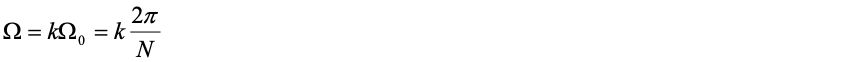进行离散化，求得其近似的频谱样值为：综上，总结对离散非周期信号（序列）频谱分析的一般求解步骤为：
（1） 确定原序列x（n）的长度M。根据能量分布，序列为无限长时需要进行截断。
（2） 确定作FFT的点数N。根据频域取样定理，为使时域波形不产生混叠，必须取NM。
（3） 使用fft命令作N点FFT计算X（k）。频率分辨率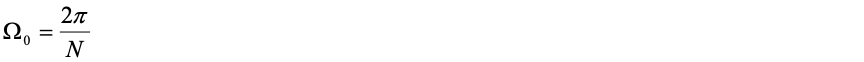（4） 绘制频谱图，使运算结果可视化。

4 实例分析及MATLAB编程
[例] 已知无限长序列x（n）=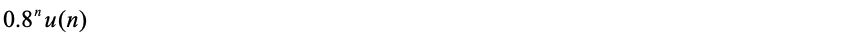（1） 截取序列长度M=10点，用FFT计算其频谱，并与理论计算的结果进行比较，观察功率泄漏现象。
（2） 截取序列长度M=20点，用FFT计算其频谱，观测功率泄漏情况并与（1）进行比较。
[解]
该序列总能量相关MATLAB运算指令：
E=1/(1-0.64)
E =
2.7778
（1）M=10点截断的信号的能量占总能量的比例通过以下MATALB指令计算：
p10=(1-(0.64).^10)/(1-0.64)/E
p10 =
0.9885
即10点截断的信号能量占原始信号总能量的98.85%，占了大部分，有少部分泄漏。
编写以下MATLAB程序观察N=M=10点时的功率泄漏现象：

N=10;
n=0:N-1;
x=(0.8).^n;
X=fft(x,N);
subplot(2,1,1);
stem(n,x);
ylabel(‘x[n]’);
xlabel(‘Time n’);
omega=2pi/Nn;
X0=1./(1-0.8exp(-jomega));
subplot(2,1,2);
plot(omega,abs(X),omega,abs(X0),‘–’);
ylabel(‘X(omega)’);

运行程序，结果显示如图4.1。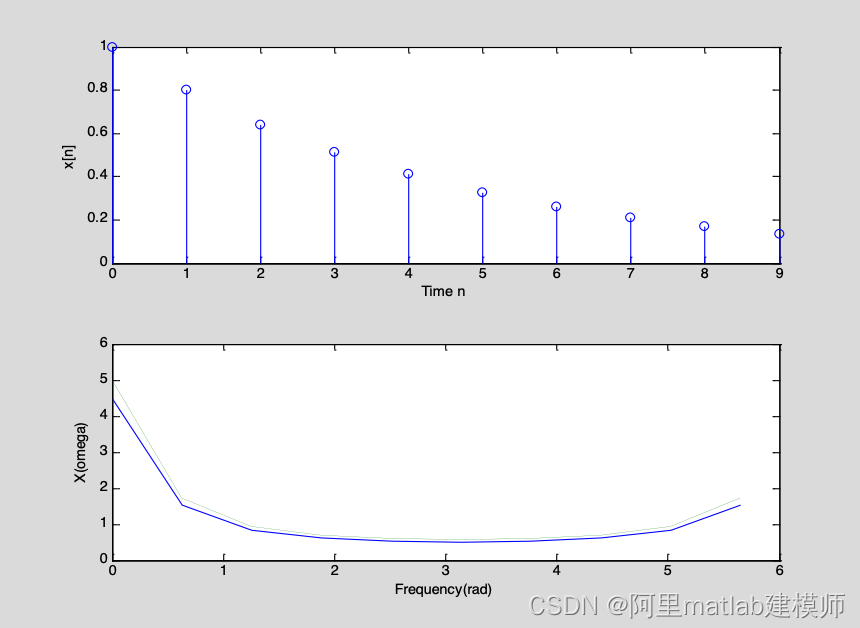图4.1  M=10点时的时域信号和DFT计算出的频谱


(2) M=20点截断的信号的能量占总能量的比例由以下MATLAB指令计算：
p20=(1-(0.64).^20)/(1-0.64)/E
p20 =
0.9999
即20点截断的信号能量占原始信号总能量的99.99%，只有0.01%的泄漏。

编写以下MATLAB程序观察N=M=20点时的功率泄漏现象：
N=20;
n=0:N-1;
x=(0.8).^n;
X=fft(x,N);
subplot(2,1,1);
stem(n,x);
ylabel(‘x[n]’);
xlabel(‘Time n’);
omega=2pi/Nn;
X0=1./(1-0.8exp(-jomega));
subplot(2,1,2);
plot(omega,abs(X),omega,abs(X0),‘–’);
ylabel(‘X(omega)’);

运行程序，结果显示如图4.2。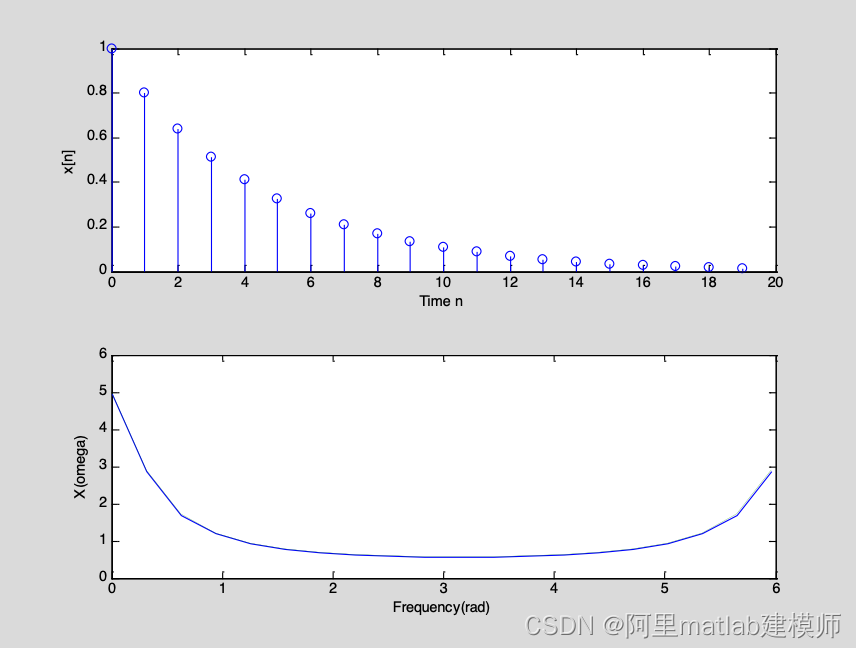4.2 M=20点时的时域信号和DFT计算出的频谱
其中，上图为截短后的时间序列，下图中实线为截短的时间序列的幅频特性，虚线为原序列的幅频特性。可见，增大M的取值后，再用FFT计算其频谱，泄露就会明显的减少，得到的谱也近似其真实谱。

5 .误差分析
DFT解决了用计算机对离散非周期信号进行分析的问题，但由于对频域是连续函数的信号需要离散化，对长度或频谱很宽或无限长的信号需要做截断处理，因此如果不能恰当地确定取样间隔T，势必产生混叠误差。如果截断和选取的长度N不合适，则会造成频谱扩散，使能量和功率产生泄露，产生泄漏误差。截断长度越短，泄漏误差也越大。为此，如何通过正确的确定T与N来减少这两种误差，实现高效计算有着极其重要的实际意义。
刚才我们从图4.1和图4.2中已经看出，时域截断对应FFT计算的频谱幅度应小与真实幅度谱，同时由于矩形窗函数对x（n）突然截断，使FFT计算的谱向高频处扩展产生了功率泄露。为了减少能量扩散，增大M的取值后，再用FFT计算其频谱，泄露就会明显的减少，得到的谱也近似其真实谱。

展开全文matlab 算法 矩阵
• 小虎最近试着将窗函数进行傅里叶变换，并利用MATLAB试着画出了它的频谱matlab 傅立叶分析 数字信号处理 c语言
• 绘制频谱图(涉利用matlab产生离散信号(周期与非周期有区别)；绘制频谱图(涉及卷积)；验证卷积定理时容易遇到的问题matlab与信号与系统的结合前言一、matlab产生一个离散的信号二、matlab算卷积绘制频谱图三、验证...
• 《用MATLAB分析离散信号的频谱与信号的采样》由会员分享，可在线阅读，更多相关《用MATLAB分析离散信号的频谱与信号的采样(7页珍藏版)》请在人人文库网上搜索。1、实验六 用MATLAB分析离散信号的频谱与信号的采样一...
• 与《时域门函数及门函数串的频谱分析》相关的范文数字信号处理综合实验报告 实验题目:基于Matlab 的语音信号去噪及仿真 专业名称: 学 号: 姓 名: 日 期: 报告内容: 一. 实验原理 1.去噪的原理 1.1 采样定理 在进行...
• 连续非周期信号频谱分析及Matlab 实现 连续非周期信号频谱分析及 MATLAB 实现 谢海霞 1，孙志雄 1 (琼州学院海南 三亚 572022) 摘要：为了便于计算机辅助计算复杂的连续信号频谱，常常采用DFT方法。DFT不仅能反映...
• 本文章简要介绍7种常见非周期信号的频谱，在信号分析中有较高的使用频率。 一、单边指数信号 表达式： 傅里叶变换： 频谱图： 二、矩形脉冲信号 表达式： 傅里叶变换： ...
• 周期信号的频谱 之前我们已经了解到了，任一满足狄利克雷条件的周期信号都可以进行傅里叶级数展开；分解成直流分量以及许多正余弦分量。 这些正余弦分量的频率必定是基频(基波频率)的整数倍，响应的频率分量分别为...
• 蓝色的线代表着连续信号的频率不断增大，竖线代表着采样点共8个，黄色的线是对采样出来的离散时间函数做的辅助线。 可以看到amazing啊！现在对它进行分析。 ----------------------------------------------------...人工智能
• 从广义上说，信号的某种特征量随...周期信号的频谱离散周期信号的单边谱：：三角型傅里叶级数 周期信号的双边谱：：指数型傅里叶级数 2. 例 【 2. 周期矩形脉冲的频谱 】 1. 原理 2. 周期频谱的关...
• 《连续传递函数离散化的方法与原理》由会员分享，可在线阅读，更多相关《连续传递函数离散化的方法与原理(33页珍藏版)》请在人人文库网上搜索。1、数字控制器的模拟化设计目录第一章 模拟化设计基础1第一节 步骤1第...
• 三角波在信号处理中具有许多应用，因为它是一种周期性的、分段线性的、连续的实函数matlab
•matlab
• 与连续信号类似，对于离散的非周期信号，可采用离散时间傅里叶变换（DTFT）进行分析。 （一）从DFS到DTFT 非周期序列可以看作周期为无穷大的周期序列，从这一思想出发，可以在周期序列的傅里叶级数DFS基础上推导非...
• 现代电子技术 ModernElectronicsTechnique 2013年6月1日 第36卷第11期 Jun.2013 Vol.36 No.11 0 引 言 频谱分析在数字信号处理中用途广泛：如滤波、检测等方面，这些都需要DFT(Discrete Fourier Transform)运算[1-3]...
• 1、周期信号的频谱离散的，非周期信号的频谱是连续的。 2、因周期信号可以用一组整数倍频率的三角函数表示，所以在频域里是离散的频率点。非周期信号做Fourier变换的时候，n趋向于无穷，所以在频谱上就变成连续的...
• 文章目录参考资料 参考资料 基波与谐波 如何使用 python 实现快速的傅里叶变换 首先对信号进行 scipy 中的 fft 变换，得到和原始信号...也可以对得到的频谱进行归一化，归一化的方式就是除以 len(fft_y) ，具体操python 开发语言
• 功率谱、频率分辨率、频谱泄漏与窗函数2008-06-01 23:49功率谱、频率分辨率、频谱泄漏与窗函数在分析和测定所采集的数据记录时，快速傅立叶变换(FFT)和功率谱是非常有用的工具。借助这些工具能够有效地采集时域信号...
• 如何确定抽样间隔 （17节，看不懂） 带限限宽 抽样定理：时域的离散化对应频域的周期化 工程应用 工程的信号是非周期函数，得到的频谱是无线延续的，不满足带限（没有最大频率），也就不能确定抽样频率。...数学
• 文章目录周期信号的频谱及特点1 周期信号的频谱2 单边谱和双边谱的关系3 周期信号频谱的特点4 周期信号的功率 周期信号的频谱及特点 频谱——信号的一种新的表示方法 1 周期信号的频谱 频谱周期信号分解后，各...
• 本文分析了用数值计算的方法实现确知连续时间信号的频谱分析，即采用离散傅里叶变换的快速算法实现对连续信号的频谱估计，然后在MATLAB语言工具下结合正弦信号给出了频谱分析的结果。论文关键词：频谱分析,离散...
• (DFT)，是傅里叶变换在时域和频域上的离散呈现形式，通俗的说就是将经过采样的有限长度时域离散采样序列变换为等长度的频域离散采样序列，通过对变换得到的频域采样序列进行适当的换算和处理，可以得到信号的频谱...matlab idft
• matlab与信号与系统的结合前言一、matlab产生一个离散的信号二、matlab算卷积绘制频谱图三、验证卷积定理四、 心得体会五、总结 前言 这真的是我第一次写博客，好吧也不是第一次，是第一次写如此高大上的博客。（咦...matlab 卷积
• 问题：对于离散信号而言，已知样点值、采样频率，如何画出与采样频率对应的幅频曲线？ 已知样点数为N，采样频率为Fs，数据记为data。则，可画出两种幅频响应： % 第一种，幅频响应频率范围为（0-Fs） Data_f = fft...matlab
• 首先回顾一下在信号与系统（10）-周期性信号的频谱中提及的方波脉冲信号，如果脉冲宽度τ\tauτ进行无线增大，则信号变为非周期信号，并且幅度频谱离散谱变为连续频谱，如下所示： 周期性方波脉冲，即： f(t)={A,&......